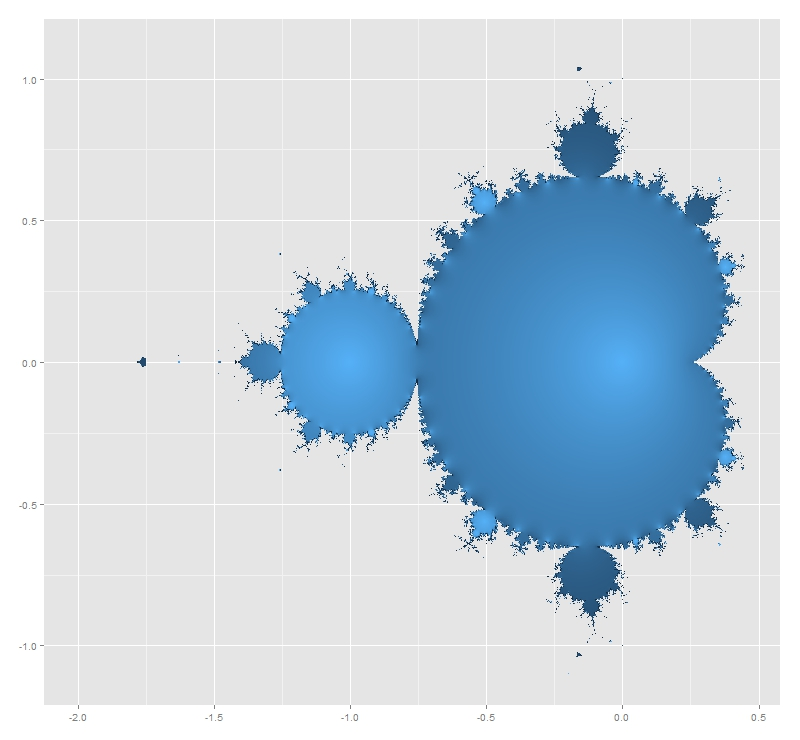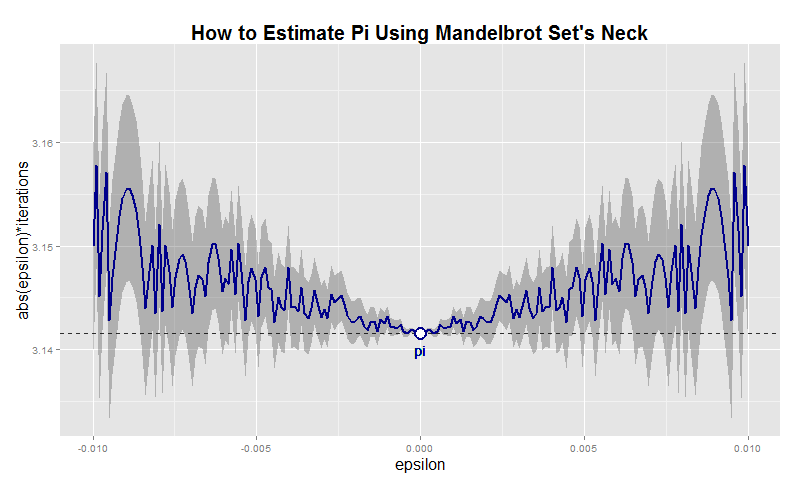# The Somnambulist and Pi

How wary we are of something warm and unborn. Something calmly by zero will divide (Unbegotten, The Somnambulist)

Some time ago, I assumed the mission to draw a plot for the cover of the new album of The Somnambulist, a music band from Berlin. They wanted a circlization of Pi, which is a graphic where numbers are represented in a circular layout. The idea is connecting each digit of Pi to its successive digit with links to the position of the numerically corresponding external sectors. I used a color palette composed by 10 nuances of the visible spectrum as a tribute for Planck, as Marco (the vocalist) requested me. After a number of attempts:The album is named Unbegotten, a german word which means archaic. As Marco told me, in theology it also means kind of eternal because of being never born and so never dying. I like how π is integrated into the title to substitute the string “tt” in the middle. Pi is also eternal so the association is genuine.

The music of The Somnambulist is intense, dark and powerful and is waiting for you here to listen it. My favorite song is the one that gives name to the album.

If you want to know more about circlizong numbers, you can visit this post, where you also can see the code I used as starting point to do this plot.

# Going Bananas #2: A Needle In A Haystack

Now I’m gonna tell my momma that I’m a traveller, I’m gonna follow the sun (The Sun, Parov Stelar)

Inspired by this book I read recently, I decided to do this experiment. The idea is comparing how easy is to find sequences of numbers inside Pi, e, Golden Ratio (Phi) and a randomly generated number. For example, since Pi is 3.1415926535897932384… the 4-size sequence 5358 can be easily found at the begining as well as the 5-size sequence 79323. I considered interesting comparing Pi with a random generated number. What I though before doing the experiment is that it would be easier finding sequences inside the andom one. Why? Because despite of being irrational and transcendental I thought there should be some kind of residual pattern in Pi that should make more difficult to find random sequences inside it than do it inside a randomly generated number.

• I downloaded Pi, e and Phi from the Internet and extract first 100.000 digits of all of them. I generate a random 100.000 number on the fly.
• I generate a representative sample of 4-size sequences
• I look for each of these sequences inside first 5.000 digits of Pi, e, Phi and the randomly generated one. I repeat searching for first 10.000, first 15.000 and so on until I search into the whole 100.000 -size number
• I store how many sequences I find for each searching
• I repeat this for 5 and 6-size sequences.

At first sight, is equally easy (or difficult), to find random sequences inside all numbers: my hypothesis was wrong.

As you can see here, 100.000 digits is more than enough to find 4-size sequences. In fact, from 45.000 digits I reach 100% of successful matches:I only find 60% of 5-size sequences inside 100.000 digits of numbers:And only 10% of 6-size sequences:Why these four numbers are so equal in order to find random sequences inside them? I don’t know. What I know is that if you want to find your telephone number inside Pi, you will probably need an enormous number of digits.

```library(rvest)
library(stringr)
library(reshape2)
library(ggplot2)
library(extrafont);windowsFonts(Comic=windowsFont("Comic Sans MS"))
library(dplyr)
library(magrittr)
library(scales)
p = html("http://www.geom.uiuc.edu/~huberty/math5337/groupe/digits.html")
e = html("http://apod.nasa.gov/htmltest/gifcity/e.2mil")
p %>%
html_text() %>%
substr(., regexpr("3.14",.), regexpr("Go to Historical",.)) %>%
gsub("[^0-9]", "", .)  %>%
substr(., 1, 100000) -> p
f %>%
html_text() %>%
substr(., regexpr("1.61",.), nchar(.)) %>%
gsub("[^0-9]", "", .) %>%
substr(., 1, 100000) -> f
e %>%
html_text() %>%
substr(., regexpr("2.71",.), nchar(.)) %>%
gsub("[^0-9]", "", .) %>%
substr(., 1, 100000) -> e
r = paste0(sample(0:9, 100000, replace = TRUE), collapse = "")
results=data.frame(Cut=numeric(0), Pi=numeric(0), Phi=numeric(0), e=numeric(0), Random=numeric(0))
bins=20
dgts=6
samp=min(10^dgts*2/100, 10000)
for (i in 1:bins) {
cut=100000/bins*i
p0=substr(p, start=0, stop=cut)
f0=substr(f, start=0, stop=cut)
e0=substr(e, start=0, stop=cut)
r0=substr(r, start=0, stop=cut)
sample(0:(10^dgts-1), samp, replace = FALSE) %>% str_pad(dgts, pad = "0") -> comb
comb %>% sapply(function(x) grepl(x, p0)) %>% sum() -> p1
comb %>% sapply(function(x) grepl(x, f0)) %>% sum() -> f1
comb %>% sapply(function(x) grepl(x, e0)) %>% sum() -> e1
comb %>% sapply(function(x) grepl(x, r0)) %>% sum() -> r1
results=rbind(results, data.frame(Cut=cut, Pi=p1, Phi=f1, e=e1, Random=r1))
}
results=melt(results, id.vars=c("Cut") , variable.name="number", value.name="matches")
opts=theme(
panel.background = element_rect(fill="darkolivegreen1"),
panel.border = element_rect(colour="black", fill=NA),
axis.line = element_line(size = 0.5, colour = "black"),
axis.ticks = element_line(colour="black"),
panel.grid.major = element_line(colour="white", linetype = 1),
panel.grid.minor = element_blank(),
axis.text.y = element_text(colour="black"),
axis.text.x = element_text(colour="black"),
text = element_text(size=20, family="Comic"),
legend.text = element_text(size=25),
legend.key = element_blank(),
legend.position = c(.75,.2),
legend.background = element_blank(),
plot.title = element_text(size = 30))
ggplot(results, aes(x = Cut, y = matches/samp, color = number))+
geom_line(size=1.5, alpha=.8)+
scale_color_discrete(name = "")+
scale_x_continuous(breaks=seq(100000/bins, 100000, by=100000/bins))+
scale_y_continuous(labels = percent)+
theme(axis.text.x = element_text(angle = 90, vjust=.5, hjust = 1))+
labs(title=paste0("Finding ",dgts, "-size strings into 100.000-digit numbers"),
x="Cut Position",
y="% of Matches")+opts
```

# What The Hell Is Pi Doing Here?

Nothing in Nature is random … A thing appears random only through the incompleteness of our knowledge (Benedict Spinoza)

This is one of my favorite mathematical mysteries. In 1991 David Boll was trying to confirm that the neck of the Mandelbrot Set is 0 in thickness. Neck is located at -0.75+0i (where two biggest circles meet each other).He tried with complex numbers like -0.75+εi for small values of ε demonstrating the divergence of all these numbers. And here comes the mystery: multiplying ε and the corresponding number of iterations it took for the iterate to diverge, gives an approximation of π that is within ±ε. Is not fascinating? I replicated David Boll’s experiment for positive and negative values of ε. I draw results as follows:Before doing it, I thought I was going to find some pattern in the graphic. Apart from the mirror effect produced by the sign of ε, there is nothing recognizable. Convergence is chaotic. Here you have the code. This example is also nice to practice with ggplot2 package, one of the totems of R:

```i<-0    # Counter of iterations
x  <- 0 # Initialization
while (Mod(x) <= 2)
{
x <- x^2+(c+complex(real = 0, imaginary = e))
i <- i+1
}
i
}
results <- as.data.frame(c(NULL,NULL))
for (j in 1:length(epsilons))
{results <- rbind(results, c(epsilons[j], testMSConvergence(epsilons[j])))}
colnames(results) <- c('epsilon', 'iterations')
dev.off()
p <- ggplot(results, aes(epsilon,abs(epsilon)*iterations))+
xlab("epsilon")+
ylab("abs(epsilon)*iterations")+
opts(axis.title.x=theme_text(size=16)) +
opts(axis.title.y=theme_text(size=16)) +
ggtitle("How to Estimate Pi Using Mandelbrot Set's Neck")+
theme(plot.title = element_text(size=20, face="bold"))
p <- p + geom_ribbon(data=results,aes(ymin=abs(epsilon)*iterations-abs(epsilon),ymax=abs(epsilon)*iterations+abs(epsilon)), alpha=0.3)
p <- p + geom_abline(intercept = pi, , slope = 0, size = 0.4, linetype=2, colour = "black", alpha=0.8)
p <- p + geom_line(colour = "dark blue", size = 1, linetype = 1)
p <- p + geom_text(x = 0, y = pi, label="pi", vjust=2, colour="dark blue")
p <- p + geom_point(x = 0, y = pi, size = 6, colour="dark blue")
p + geom_point(x = 0, y = pi, size = 4, colour="white")
```